• 一维卷积神经网络（1D CNNs）只在一定程度上有所涉及，比如在自然语言处理（NLP）中的应用。目前很少有文章能够提供关于如何构造一维卷积神经网络来解决你可能正面临的一些机器学习问题。本文试图补上这样一个短板...

为了方便读者理解一维卷积应用，请配合笔者的实战文章一起食用：

1.[深度应用]·首届中国心电智能大赛初赛开源Baseline（基于Keras val_acc: 0.88）

2.[深度应用]·DC竞赛轴承故障检测开源Baseline（基于Keras1D卷积 val_acc:0.99780）

3.https://github.com/xiaosongshine/bearing_detection_by_conv1d

4.『带你学AI』带你学AI与TensorFlow2实战之入门初探：如何速成深度学习开发

5.笔者深度学公众号《简明AI》：

概述

许多技术文章都关注于二维卷积神经网络（2D CNN）的使用，特别是在图像识别中的应用。而一维卷积神经网络（1D CNNs）只在一定程度上有所涉及，比如在自然语言处理（NLP）中的应用。目前很少有文章能够提供关于如何构造一维卷积神经网络来解决你可能正面临的一些机器学习问题。

本文试图补上这样一个短板。

何时应用 1D CNN？

CNN 可以很好地识别出数据中的简单模式，然后使用这些简单模式在更高级的层中生成更复杂的模式。当你希望从整体数据集中较短的（固定长度）片段中获得感兴趣特征，并且该特性在该数据片段中的位置不具有高度相关性时，1D CNN 是非常有效的。

1D CNN 可以很好地应用于传感器数据的时间序列分析（比如陀螺仪或加速度计数据）；同样也可以很好地用于分析具有固定长度周期的信号数据（比如音频信号）。此外，它还能应用于自然语言处理的任务（由于单词的接近性可能并不总是一个可训练模式的好指标，因此 LSTM 网络在 NLP 中的应用更有前途）。

1D CNN 和 2D CNN 之间有什么区别？

无论是一维、二维还是三维，卷积神经网络（CNNs）都具有相同的特点和相同的处理方法。关键区别在于输入数据的维数以及特征检测器（或滤波器）如何在数据之间滑动：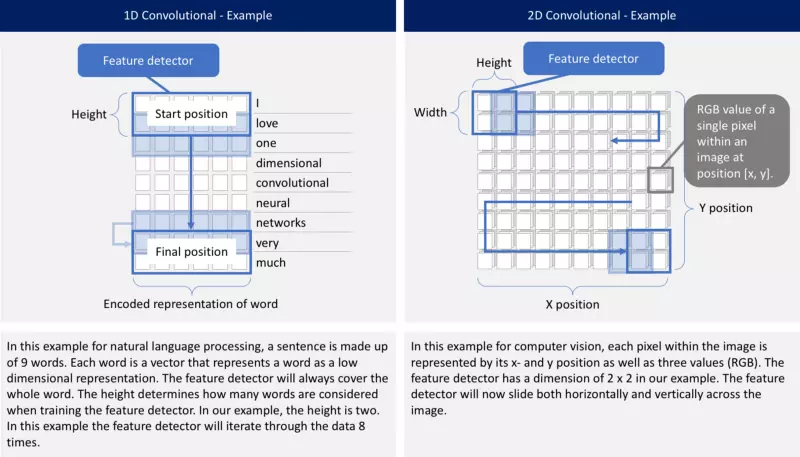问题描述

在本文中，我们将专注于基于时间片的加速度传感器数据的处理，这些数据来自于用户的腰带式智能手机设备。基于 x、y 和 z 轴的加速度计数据，1D CNN 用来预测用户正在进行的活动类型（比如“步行”、“慢跑”或“站立”）。你可以在我的另外两篇文章中找到更多的信息 这里 和 这里。对于各种活动，在每个时间间隔上的数据看起来都与此类似。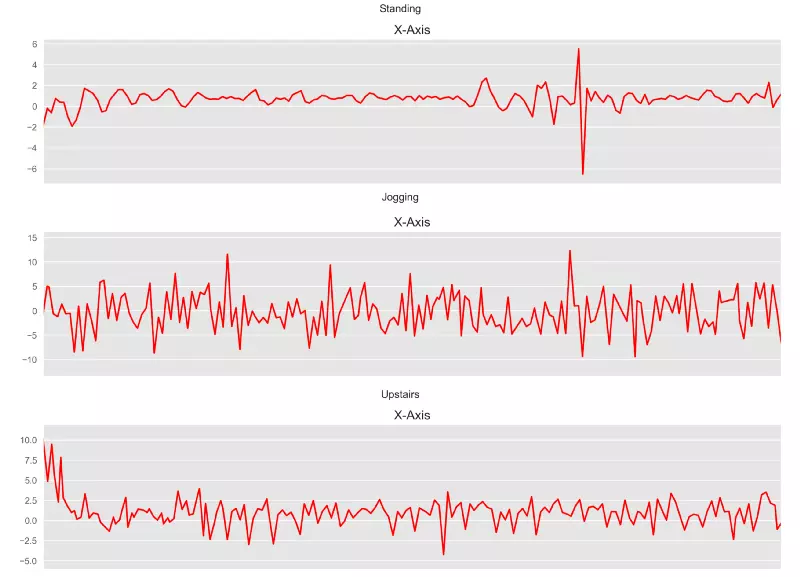来自加速度计数据的时间序列样例

如何在 PYTHON 中构造一个 1D CNN？

目前已经有许多得标准 CNN 模型可用。我选择了 Keras 网站 上描述的一个模型，并对它进行了微调，以适应前面描述的问题。下面的图片对构建的模型进行一个高级概述。其中每一层都将会进一步加以解释。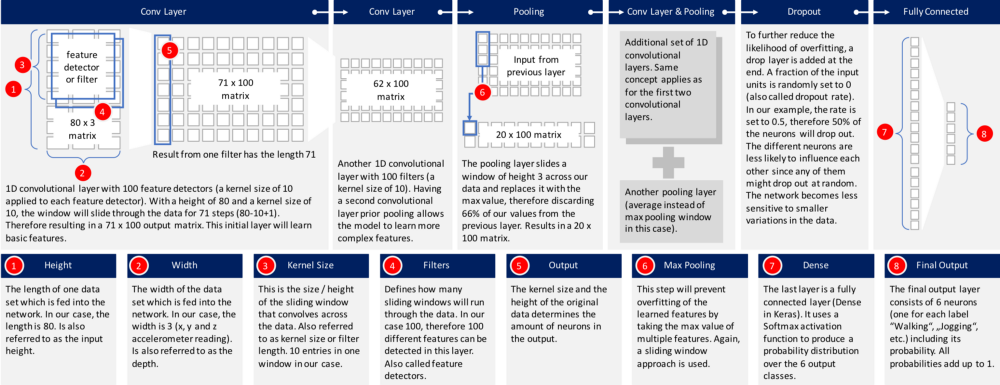让我们先来看一下对应的 Python 代码，以便构建这个模型：

model_m = Sequential()
print(model_m.summary())

运行这段代码将得到如下的深层神经网络：

_________________________________________________________________
Layer (type)                 Output Shape              Param #
=================================================================
reshape_45 (Reshape)         (None, 80, 3)             0
_________________________________________________________________
conv1d_145 (Conv1D)          (None, 71, 100)           3100
_________________________________________________________________
conv1d_146 (Conv1D)          (None, 62, 100)           100100
_________________________________________________________________
max_pooling1d_39 (MaxPooling (None, 20, 100)           0
_________________________________________________________________
conv1d_147 (Conv1D)          (None, 11, 160)           160160
_________________________________________________________________
conv1d_148 (Conv1D)          (None, 2, 160)            256160
_________________________________________________________________
global_average_pooling1d_29  (None, 160)               0
_________________________________________________________________
dropout_29 (Dropout)         (None, 160)               0
_________________________________________________________________
dense_29 (Dense)             (None, 6)                 966
=================================================================
Total params: 520,486
Trainable params: 520,486
Non-trainable params: 0
_________________________________________________________________
None

让我们深入到每一层中，看看到底发生了什么：

输入数据： 数据经过预处理后，每条数据记录中包含有 80 个时间片（数据是以 20Hz 的采样频率进行记录的，因此每个时间间隔中就包含有 4 秒的加速度计数据）。在每个时间间隔内，存储加速度计的 x 轴、 y 轴和 z 轴的三个数据。这样就得到了一个 80 x 3 的矩阵。由于我通常是在 iOS 系统中使用神经网络的，所以数据必须平展成长度为 240 的向量后传入神经网络中。网络的第一层必须再将其变形为原始的 80 x 3 的形状。

第一个 1D CNN 层： 第一层定义了高度为 10（也称为卷积核大小）的滤波器（也称为特征检测器）。只有定义了一个滤波器，神经网络才能够在第一层中学习到一个单一的特征。这可能还不够，因此我们会定义 100 个滤波器。这样我们就在网络的第一层中训练得到 100 个不同的特性。第一个神经网络层的输出是一个 71 x 100 的矩阵。输出矩阵的每一列都包含一个滤波器的权值。在定义内核大小并考虑输入矩阵长度的情况下，每个过滤器将包含 71 个权重值。

第二个 1D CNN 层： 第一个 CNN 的输出结果将被输入到第二个 CNN 层中。我们将在这个网络层上再次定义 100 个不同的滤波器进行训练。按照与第一层相同的逻辑，输出矩阵的大小为 62 x 100。

最大值池化层： 为了减少输出的复杂度和防止数据的过拟合，在 CNN 层之后经常会使用池化层。在我们的示例中，我们选择了大小为 3 的池化层。这意味着这个层的输出矩阵的大小只有输入矩阵的三分之一。

第三和第四个 1D CNN 层： 为了学习更高层次的特征，这里又使用了另外两个 1D CNN 层。这两层之后的输出矩阵是一个 2 x 160 的矩阵。

平均值池化层： 多添加一个池化层，以进一步避免过拟合的发生。这次的池化不是取最大值，而是取神经网络中两个权重的平均值。输出矩阵的大小为 1 x 160 。每个特征检测器在神经网络的这一层中只剩下一个权重。

Dropout 层： Dropout 层会随机地为网络中的神经元赋值零权重。由于我们选择了 0.5 的比率，则 50% 的神经元将会是零权重的。通过这种操作，网络对数据的微小变化的响应就不那么敏感了。因此，它能够进一步提高对不可见数据处理的准确性。这个层的输出仍然是一个 1 x 160 的矩阵。

使用 Softmax 激活的全连接层： 最后一层将会把长度为 160 的向量降为长度为 6 的向量，因为我们有 6 个类别要进行预测（即 “慢跑”、“坐下”、“走路”、“站立”、“上楼”、“下楼”）。这里的维度下降是通过另一个矩阵乘法来完成的。Softmax 被用作激活函数。它强制神经网络的所有六个输出值的加和为一。因此，输出值将表示这六个类别中的每个类别出现的概率。

训练和测试该神经网络

下面是一段用以训练模型的 Python 代码，批大小为 400，其中训练集和验证集的分割比例是 80 比 20。

callbacks_list = [
keras.callbacks.ModelCheckpoint(
filepath='best_model.{epoch:02d}-{val_loss:.2f}.h5',
monitor='val_loss', save_best_only=True),
keras.callbacks.EarlyStopping(monitor='acc', patience=1)
]

model_m.compile(loss='categorical_crossentropy',

BATCH_SIZE = 400
EPOCHS = 50

history = model_m.fit(x_train,
y_train,
batch_size=BATCH_SIZE,
epochs=EPOCHS,
callbacks=callbacks_list,
validation_split=0.2,
verbose=1)

该模型在训练数据上的准确率可达 97%。

...
Epoch 9/50
16694/16694 [==============================] - 16s 973us/step - loss: 0.0975 - acc: 0.9683 - val_loss: 0.7468 - val_acc: 0.8031
Epoch 10/50
16694/16694 [==============================] - 17s 989us/step - loss: 0.0917 - acc: 0.9715 - val_loss: 0.7215 - val_acc: 0.8064
Epoch 11/50
16694/16694 [==============================] - 17s 1ms/step - loss: 0.0877 - acc: 0.9716 - val_loss: 0.7233 - val_acc: 0.8040
Epoch 12/50
16694/16694 [==============================] - 17s 1ms/step - loss: 0.0659 - acc: 0.9802 - val_loss: 0.7064 - val_acc: 0.8347
Epoch 13/50
16694/16694 [==============================] - 17s 1ms/step - loss: 0.0626 - acc: 0.9799 - val_loss: 0.7219 - val_acc: 0.8107

根据测试集数据进行测试，其准确率为 92%。

Accuracy on test data: 0.92

Loss on test data: 0.39

总结

本文通过以智能手机的加速度计数据来预测用户的行为为例，绍了如何使用 1D CNN 来训练网络。

补充阅读

1.[深度应用]·首届中国心电智能大赛初赛开源Baseline（基于Keras val_acc: 0.88）

2.[深度应用]·DC竞赛轴承故障检测开源Baseline（基于Keras1D卷积 val_acc:0.99780）

3.https://github.com/xiaosongshine/bearing_detection_by_conv1d

欢迎Fork+Star，觉得有用的话，麻烦小小鼓励一下 ><

欢迎大家关注小宋公众号《简明AI》带你学深度学习：这里收集了一些比较适合入门实战的PDF书籍，覆盖TensorFlow、PyTorch与MXNet。推荐的理由是通俗易懂，适合初学者研究学习。书籍列表如下：《简单粗暴TensorFlow2最新中文版》《动手学深度学习PyTorch最新中文版》《动手学深度学习MXNet最新中文版》
关注后，回复：书籍，领取。基于深度学习的理论学习与应用开发技术分享，笔者会经常分享深度学习干货内容，大家在学习或者应用深度学习时，遇到什么问题也可以与我在上面交流知无不答。

出自CSDN博客专家&知乎深度学习专栏作家@小宋是呢


展开全文• ## 【keras】一维卷积神经网络多分类

万次阅读 多人点赞 2020-06-20 13:05:05
刚刚接触到深度学习，前2个月的时间里，我用一维的卷积神经网络实现了对于一维数据集的分类和回归。由于在做这次课题之前，我对深度学习基本上没有过接触，所以期间走了很多弯路。 在刚刚收到题目的要求时，我选择...
刚刚接触到深度学习，前2个月的时间里，我用一维的卷积神经网络实现了对于一维数据集的分类和回归。由于在做这次课题之前，我对深度学习基本上没有过接触，所以期间走了很多弯路。

在刚刚收到题目的要求时，我选择使用TensorFlow来直接编写函数，结果由于没有什么基础，写了一个周我就放弃了，改用keras来完成我的任务。

用keras来搭建神经网络其实很简单。我把自己的网络模型和数据集分享给大家，一起交流一起进步。

我们要完成的任务是对一些给定的湿度特征数据进行分类，多分类就是最简单最入门的深度学习案例。

我们要完成分类任务的数据集，247*900大小的一个矩阵，其中包含900条湿度信息，每条湿度信息由246位比特构成，最后一位表示湿度的类别，数值1-9，也就是每种湿度有100条。大家可以点击下边的链接自取。

数据集-用做分类.csv

或者百度网盘：

链接：https://pan.baidu.com/s/1R0Ok5lB_RaI2cVHychZuxQ
提取码：9nwi
复制这段内容后打开百度网盘手机App，操作更方便哦

首先是数据的导入：

# 载入数据
X = np.expand_dims(df.values[:, 0:246].astype(float), axis=2)
Y = df.values[:, 246]

X是900条数据的特征信息，Y表示真实类别。特别注意：这里的X在读取的时候矩阵增加了一维。使用一维卷积神经网络Conv1D的时候必须要事先这样对数据集进行处理，而只使用深度网络Dense时不需要额外的增加一维。

具体细节大家可以看我之前写过的一篇博客，比较细的区分了这两种情况：

Conv1D层与Dense层的连接

没有接触过的读者可以查一下只使用深度层和使用卷积层的区别。CNN眼里只有局部小特征，而DNN只有全局大特征。

最普通的深层神经网络包含多层神经元，从输入信号中提取信息。每个神经元接受来自前一层神经元的输入，并通过权重和非线性将它们组合起来。与普通神经网络中每个神经元与前一层的所有神经元连接不同，CNN中的每个神经元只与前一层的少数神经元局部连接。而且，CNN同一层的所有神经元都有相同的权重。CNN层中神经元的值通常被称为特征映射（features maps），因为它们可以被看作是对应于输入的不同部分的特征。卷积神经网络可以很好地捕获出原数据中的局部简单特征，随着层数的增加，产生的特征映射会捕获输入信号越来越多的全局和更复杂的属性。它可以在一条数据中较短的片段中获得感兴趣的特征，并且该特征在该数据片段中的位置不具有高度相关性

紧接着是对湿度类别的编码：

# 湿度分类编码为数字
encoder = LabelEncoder()
Y_encoded = encoder.fit_transform(Y)
Y_onehot = np_utils.to_categorical(Y_encoded)

Y_onehot编码是为了让程序自己来区分每一条特征信息而添加的编码。一个简单的例子解释一下：

这一步转化工作我们可以利用keras中的np_utils.to_categorical函数来进行。

“one-hot编码。one-hot编码的定义是用N位状态寄存器来对N个状态进行编码。比如[0,...,0]，[1,...,1]，[2,...,2]有3个分类值,因此N为3,对应的one-hot编码可以表示为100,010,001。”

我们将原始的数据集划分为训练集和测试集：

# 划分训练集，测试集
X_train, X_test, Y_train, Y_test = train_test_split(X, Y_onehot, test_size=0.3, random_state=0)

我这里把原始数据集以7：3的比例划分为训练集和测试集。训练集用来逼近真实类别，测试集来测试效果。

接着的是网络模型的搭建：

# 定义神经网络
def baseline_model():
model = Sequential()
plot_model(model, to_file='./model_classifier.png', show_shapes=True) # 保存网络结构为图片，这一步可以直接去掉
print(model.summary()) # 显示网络结构
return model

这一步往往是最核心的一步，网络模型通过卷积层来提取特征，在分类任务中，网络的最后一层为每个类。分类算法得到的是一个决策面，通过对比湿度信息属于每一类湿度的概率，进而对号入座。

经过多次调参尝试，最后我使用7层Conv1D来提取特征值，每两层Conv1D后添加一层MaxPooling1D来保留主要特征，减少计算量。每层卷积层使用双曲正切函数tanh（hyperbolic tangent function）来提高神经网络对模型的表达能力。tanh经常被运用到多分类任务中用做激活函数。

神经网络本就具有不可解释性，一般卷积核和全连接的结点数按照2的指数次幂来取。

Flatten()层作为中间层来链接卷积神经网络和全连接层。

神经网络被训练来最小化一个损失函数（Softmax loss），该函数捕捉到网络的特征输出与给定的期望输出之间的差异。9个输出结点对应九个类别的湿度，Softmax将每个特征数据匹配到概率最大的特征类别。

交叉熵损失函数（categorical crossentropy）作为模型训练的损失函数，它刻画的是当前学习到的概率分布与实际概率分布的距离，也就是损失函数越小，两个概率分布越相似，此时损失函数接近于0。

我将网络模型绘制成了AlexNet风格的示意图：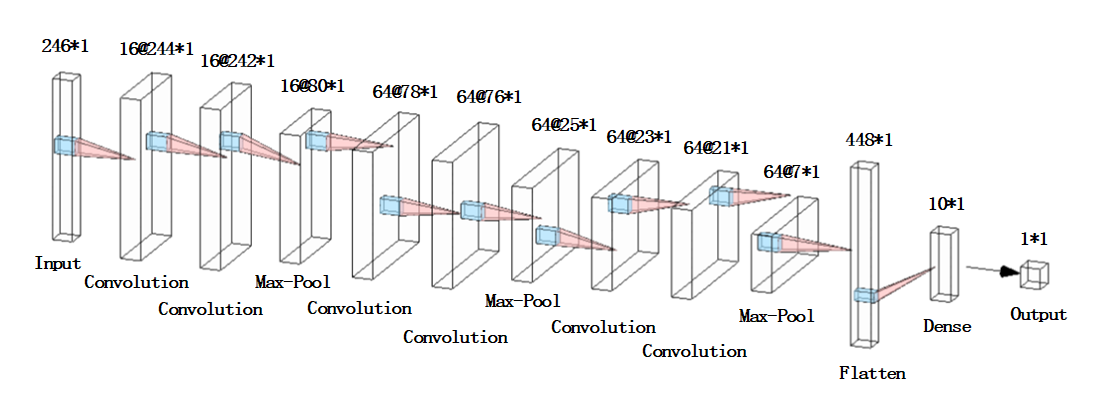这里也给大家安利下这个很好用的绘制神经网络的工具：

http://alexlenail.me/NN-SVG/AlexNet.html

搭建好网络之后就可以开始训练了。

# 训练分类器
estimator = KerasClassifier(build_fn=baseline_model, epochs=40, batch_size=1, verbose=1)
estimator.fit(X_train, Y_train)

batch_size设为1，然后一共训练40期。

这些数据大家都可以根据自己的实际情况做出调整和优化。

到这一步已经是搭建和训练的部分全部结束了。

紧接着是测试集来验证训练的准确性。

为了每次测试前不重复训练，我们将训练的模型保存下来：

# 将其模型转换为json
model_json = estimator.model.to_json()
with open(r"C:\Users\Desktop\model.json",'w')as json_file:
json_file.write(model_json)# 权重不在json中,只保存网络结构
estimator.model.save_weights('model.h5')

下面是读取模型的部分，这一部分完全可以写到另一个函数里：

# 加载模型用做预测
json_file = open(r"C:\Users\Desktop\model.json", "r")
json_file.close()
# 分类准确率
print("The accuracy of the classification model:")
print('%s: %.2f%%' % (loaded_model.metrics_names, scores * 100))
# 输出预测类别
print("predicted label:\n " + str(predicted_label))

当然我们也希望可以直观的显示出模型的分类精度，和训练的过程。

混淆矩阵经常用来表示分类的效果。

#显示混淆矩阵
plot_confuse(estimator.model, X_test, Y_test)

当然也需要我们自己写出来：

# 混淆矩阵定义
def plot_confusion_matrix(cm, classes,title='Confusion matrix',cmap=plt.cm.jet):
cm = cm.astype('float') / cm.sum(axis=1)[:, np.newaxis]
plt.imshow(cm, interpolation='nearest', cmap=cmap)
plt.rcParams['font.sans-serif'] = ['SimHei']  # 用来正常显示中文标签
plt.rcParams['axes.unicode_minus'] = False  # 用来正常显示负号
plt.colorbar()
tick_marks = np.arange(len(classes))
plt.xticks(tick_marks,('0%','3%','5%','8%','10%','12%','15%','18%','20%','25%'))
plt.yticks(tick_marks,('0%','3%','5%','8%','10%','12%','15%','18%','20%','25%'))
thresh = cm.max() / 2.
for i, j in itertools.product(range(cm.shape), range(cm.shape)):
plt.text(j, i, '{:.2f}'.format(cm[i, j]), horizontalalignment="center",color="white" if cm[i, j] > thresh else "black")
plt.tight_layout()
plt.ylabel('真实类别')
plt.xlabel('预测类别')
plt.savefig('test_xx.png', dpi=200, bbox_inches='tight', transparent=False)
plt.show()

# 显示混淆矩阵
def plot_confuse(model, x_val, y_val):
predictions = model.predict_classes(x_val)
truelabel = y_val.argmax(axis=-1)   # 将one-hot转化为label
conf_mat = confusion_matrix(y_true=truelabel, y_pred=predictions)
plt.figure()
plot_confusion_matrix(conf_mat, range(np.max(truelabel)+1))


如果好奇的话你也可以看看每层卷积层到底学到了什么：

# 可视化卷积层
visual(estimator.model, X_train, 1)

但是我觉得看一维卷积层的特征没啥意义，毕竟都是一些点，看起来有点非人类。

# 卷积网络可视化
def visual(model, data, num_layer=1):
# data:图像array数据
# layer:第n层的输出
layer = keras.backend.function([model.layers.input], [model.layers[num_layer].output])
f1 = layer([data])
print(f1.shape)
num = f1.shape[-1]
print(num)
plt.figure(figsize=(8, 8))
for i in range(num):
plt.subplot(np.ceil(np.sqrt(num)), np.ceil(np.sqrt(num)), i+1)
plt.imshow(f1[:, :, i] * 255, cmap='gray')
plt.axis('off')
plt.show()

最后运行的所有结果如下：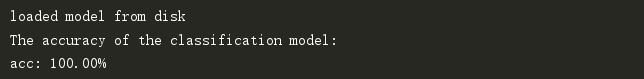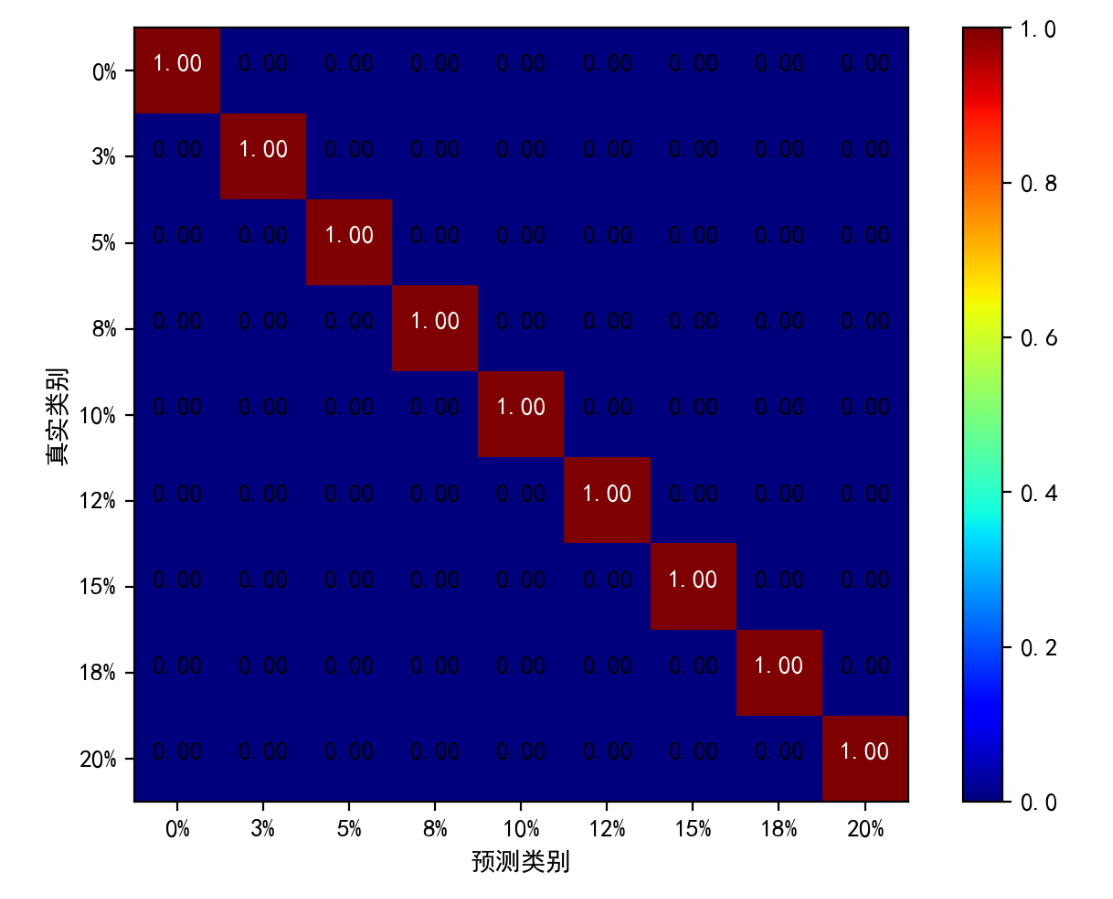这个结果这么好纯粹是因为数据比较好，分类的准确率很大程度都是依赖你数据集本身的区分度的。

下面是数据集的特征图，从图上就可以看出数据的层次性很直观。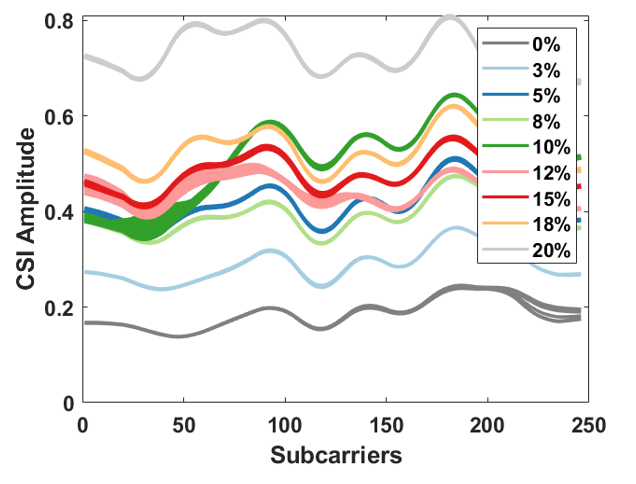当然我给大家上传的这部分数据是我这边结果最好的一批，其他数据集准确度达不到这么高。

下面贴出整个分类过程的完整代码：

# -*- coding: utf8 -*-
import numpy as np
import pandas as pd
import keras
from keras.models import Sequential
from keras.wrappers.scikit_learn import KerasClassifier
from keras.utils import np_utils,plot_model
from sklearn.model_selection import cross_val_score,train_test_split,KFold
from sklearn.preprocessing import LabelEncoder
from keras.layers import Dense,Dropout,Flatten,Conv1D,MaxPooling1D
from keras.models import model_from_json
import matplotlib.pyplot as plt
from sklearn.metrics import confusion_matrix
import itertools

# 载入数据
X = np.expand_dims(df.values[:, 0:246].astype(float), axis=2)
Y = df.values[:, 246]

# 湿度分类编码为数字
encoder = LabelEncoder()
Y_encoded = encoder.fit_transform(Y)
Y_onehot = np_utils.to_categorical(Y_encoded)

# 划分训练集，测试集
X_train, X_test, Y_train, Y_test = train_test_split(X, Y_onehot, test_size=0.3, random_state=0)

# 定义神经网络
def baseline_model():
model = Sequential()
plot_model(model, to_file='./model_classifier.png', show_shapes=True)
print(model.summary())
return model

# 训练分类器
estimator = KerasClassifier(build_fn=baseline_model, epochs=40, batch_size=1, verbose=1)
estimator.fit(X_train, Y_train)

# 卷积网络可视化
# def visual(model, data, num_layer=1):
#     # data:图像array数据
#     # layer:第n层的输出
#     layer = keras.backend.function([model.layers.input], [model.layers[num_layer].output])
#     f1 = layer([data])
#     print(f1.shape)
#     num = f1.shape[-1]
#     print(num)
#     plt.figure(figsize=(8, 8))
#     for i in range(num):
#         plt.subplot(np.ceil(np.sqrt(num)), np.ceil(np.sqrt(num)), i+1)
#         plt.imshow(f1[:, :, i] * 255, cmap='gray')
#         plt.axis('off')
#     plt.show()

# 混淆矩阵定义
def plot_confusion_matrix(cm, classes,title='Confusion matrix',cmap=plt.cm.jet):
cm = cm.astype('float') / cm.sum(axis=1)[:, np.newaxis]
plt.imshow(cm, interpolation='nearest', cmap=cmap)
plt.rcParams['font.sans-serif'] = ['SimHei']  # 用来正常显示中文标签
plt.rcParams['axes.unicode_minus'] = False  # 用来正常显示负号
plt.colorbar()
tick_marks = np.arange(len(classes))
plt.xticks(tick_marks,('0%','3%','5%','8%','10%','12%','15%','18%','20%','25%'))
plt.yticks(tick_marks,('0%','3%','5%','8%','10%','12%','15%','18%','20%','25%'))
thresh = cm.max() / 2.
for i, j in itertools.product(range(cm.shape), range(cm.shape)):
plt.text(j, i, '{:.2f}'.format(cm[i, j]), horizontalalignment="center",color="white" if cm[i, j] > thresh else "black")
plt.tight_layout()
plt.ylabel('真实类别')
plt.xlabel('预测类别')
plt.savefig('test_xx.png', dpi=200, bbox_inches='tight', transparent=False)
plt.show()

# seed = 42
# np.random.seed(seed)
# kfold = KFold(n_splits=10, shuffle=True, random_state=seed)
# result = cross_val_score(estimator, X, Y_onehot, cv=kfold)
# print("Accuracy of cross validation, mean %.2f, std %.2f\n" % (result.mean(), result.std()))

# 显示混淆矩阵
def plot_confuse(model, x_val, y_val):
predictions = model.predict_classes(x_val)
truelabel = y_val.argmax(axis=-1)   # 将one-hot转化为label
conf_mat = confusion_matrix(y_true=truelabel, y_pred=predictions)
plt.figure()
plot_confusion_matrix(conf_mat, range(np.max(truelabel)+1))

# 将其模型转换为json
model_json = estimator.model.to_json()
with open(r"C:\Users\316CJW\Desktop\毕设代码\model.json",'w')as json_file:
json_file.write(model_json)# 权重不在json中,只保存网络结构
estimator.model.save_weights('model.h5')

# 加载模型用做预测
json_file = open(r"C:\Users\Desktop\model.json", "r")
json_file.close()
# 分类准确率
print("The accuracy of the classification model:")
print('%s: %.2f%%' % (loaded_model.metrics_names, scores * 100))
# 输出预测类别
print("predicted label:\n " + str(predicted_label))
#显示混淆矩阵
plot_confuse(estimator.model, X_test, Y_test)

# 可视化卷积层
# visual(estimator.model, X_train, 1)

但是，湿度值到底是连续的数值，分类任务验证了我们数据集特征的可分辨性。

下一篇博客中，我将对数据集稍作修改，将湿度类别改为真实湿度值。

利用卷积神经网络来提取特征，实现线性回归，二者同出一脉。

【keras】一维卷积神经网络做回归

比起其他科普的博客，我这篇文章更像是在学习如何利用工具做深度学习。

希望大家多多支持，不足之处互相交流(●ˇ∀ˇ●)！


展开全文深度学习 分类算法 python
• ## 【keras】一维卷积神经网络做回归

千次阅读 多人点赞 2020-06-20 13:51:50
【keras】一维卷积神经网络多分类 在这篇博客当中我将利用一个稍加修改的数据集来完成线性回归任务。相比较以往的线性回归处理方式，我认为使用神经网络实现线性回归要简单和准确得多。 数据集大小仍然是247*900，...
在上一篇博客里我介绍了如何利用keras对一个给定的数据集来完成多分类任务。

100%的分类准确度验证了分类模型的可行性和数据集的准确度。

【keras】一维卷积神经网络多分类

在这篇博客当中我将利用一个稍加修改的数据集来完成线性回归任务。相比较以往的线性回归处理方式，我认为使用神经网络实现线性回归要简单和准确得多。

数据集大小仍然是247*900，不同的是数据集的第247位变成了湿度特征的真实湿度值。

用来表示湿度的样本是我们自己配置的，所以真实的湿度都是有理可循的，不是为了突出不同类别而捏造的。

数据集-用做回归.csv

或者百度网盘：

链接：https://pan.baidu.com/s/1R0Ok5lB_RaI2cVHychZuxQ
提取码：9nwi
复制这段内容后打开百度网盘手机App，操作更方便哦

不同于分类算法得到的决策面，回归算法得到的是一个最优拟合线，这个线条可以最好的接近数据集中得各个点。

首先依旧是数据集的导入和划分：

# 载入数据
X = np.expand_dims(df.values[:, 0:246].astype(float), axis=2)#增加一维轴
Y = df.values[:, 246]

# 划分训练集，测试集
X_train, X_test, Y_train, Y_test = train_test_split(X, Y, test_size=0.5, random_state=0)

这里我便不做细说了，想要了解的同学可以看一下上一篇博客。

接着是网络模型的搭建：

# 定义一个神经网络
model = Sequential()
plot_model(model, to_file='./model_linear.png', show_shapes=True)
print(model.summary())
model.compile(optimizer='adam', loss='mean_squared_error', metrics=[coeff_determination])

为了完成回归任务，神经网络的输出层需要被设置为一个结点，它表示输出每一条湿度信息的预测结果。

model.add(Dense(1, activation='linear'))

我们使用均方误差（Mean Squared Error，MSE）做输出层的损失函数，MSE经常被用做来比较模型预测值与真实值的偏差，在我们的任务中，通过不断减小损失函数的值，进而让整个网络尽可能地去拟合它真实的湿度值。

整个网络模型的示意图如下：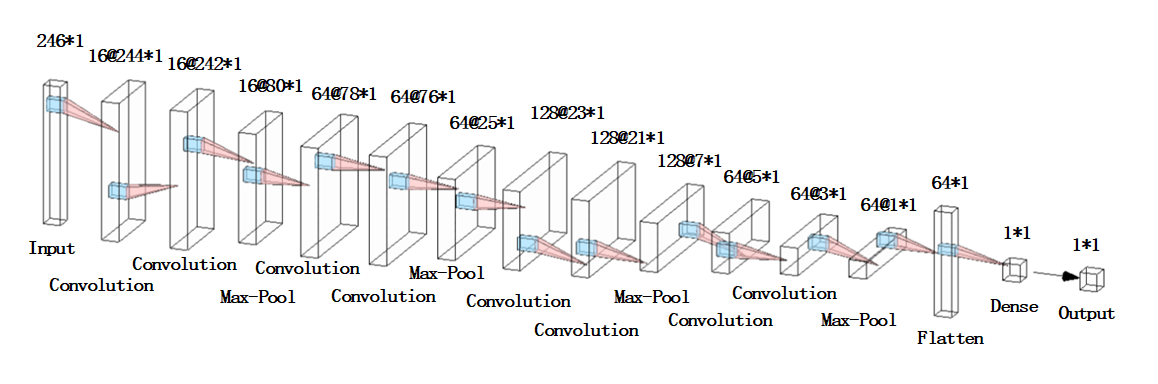经过多次调参之后，我们选用8层Conv1D来提取特征，每两层Conv1D后添加一层MaxPooling1D来保留主要特征，减少计算量。每层卷积层使用线性整流函数（Rectified Linear Unit, ReLU）作为激活函数。最后一层深度层输出湿度预测值，在MSE损失函数的逼近下，湿度的预测值会愈来愈趋向于真实值。

为了可以更准确的回归数据的真实湿度值，使用的网络层数明显比分类时要更深。

为了评估网络模型训练和测试过程的准确度，我们需要自定义度量函数：

决定系数R2（coefficient ofdetermination）常常在线性回归中被用来表征有多少百分比的因变量波动被回归线描述。如果R2 =1则表示模型完美地预测了目标变量。

表达式：R2=SSR/SST=1-SSE/SST
其中：SST=SSR+SSE，SST(total sum of squares)为总平方和，SSR(regression sum of squares)为回归平方和，SSE(error sum of squares) 为残差平方和。

# 自定义度量函数
def coeff_determination(y_true, y_pred):
SS_res =  K.sum(K.square( y_true-y_pred ))
SS_tot = K.sum(K.square( y_true - K.mean(y_true) ) )
return ( 1 - SS_res/(SS_tot + K.epsilon()) )

并把它运用到编译中来：

model.compile(optimizer='adam', loss='mean_squared_error', metrics=[coeff_determination])

下面贴出整个运行过程的完整代码：

# -*- coding: utf8 -*-
import numpy as np
import pandas as pd
from keras.models import Sequential
from keras.utils import np_utils,plot_model
from sklearn.model_selection import cross_val_score,train_test_split
from keras.layers import Dense, Dropout,Flatten,Conv1D,MaxPooling1D
from keras.models import model_from_json
import matplotlib.pyplot as plt
from keras import backend as K

# 载入数据
X = np.expand_dims(df.values[:, 0:246].astype(float), axis=2)#增加一维轴
Y = df.values[:, 246]

# 划分训练集，测试集
X_train, X_test, Y_train, Y_test = train_test_split(X, Y, test_size=0.5, random_state=0)

# 自定义度量函数
def coeff_determination(y_true, y_pred):
SS_res =  K.sum(K.square( y_true-y_pred ))
SS_tot = K.sum(K.square( y_true - K.mean(y_true) ) )
return ( 1 - SS_res/(SS_tot + K.epsilon()) )

# 定义一个神经网络
model = Sequential()
plot_model(model, to_file='./model_linear.png', show_shapes=True)
print(model.summary())

# 训练模型
model.fit(X_train,Y_train, validation_data=(X_test, Y_test),epochs=40, batch_size=10)

# # 将其模型转换为json
# model_json = model.to_json()
# with open(r"C:\Users\Desktop\model.json",'w')as json_file:
#     json_file.write(model_json)# 权重不在json中,只保存网络结构
# model.save_weights('model.h5')
#
# # 加载模型用做预测
# json_file = open(r"C:\Users\Desktop\model.json", "r")
# json_file.close()
# scores = model.evaluate(X_test,Y_test,verbose=0)
# print('%s: %.2f%%' % (model.metrics_names, scores*100))

# 准确率
scores = model.evaluate(X_test,Y_test,verbose=0)
print('%s: %.2f%%' % (model.metrics_names, scores*100))

# 预测值散点图
predicted = model.predict(X_test)
plt.scatter(Y_test,predicted)
x=np.linspace(0,0.3,100)
y=x
plt.plot(x,y,color='red',linewidth=1.0,linestyle='--',label='line')
plt.rcParams['font.sans-serif'] = ['SimHei']  # 用来正常显示中文标签
plt.rcParams['axes.unicode_minus'] = False  # 用来正常显示负号
plt.legend(["y = x","湿度预测值"])
plt.title("预测值与真实值的偏离程度")
plt.xlabel('真实湿度值')
plt.ylabel('湿度预测值')
plt.savefig('test_xx.png', dpi=200, bbox_inches='tight', transparent=False)
plt.show()

# 计算误差
result =abs(np.mean(predicted - Y_test))
print("The mean error of linear regression:")
print(result)

在评估实验结果时，我是输出了决定系数的值以及回归湿度和真实湿度的平均偏差：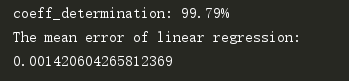可以看出99%的点找到了他们应该去的归宿，即被回归线所扫瞄到。

平均误差在0.0014，可以说是一个很好的结果。

另一方面，我以真实湿度为x轴，预测湿度为y轴绘制了预测数据的散点图。

从图中可以看出预测数据较好的逼近了真实湿度值。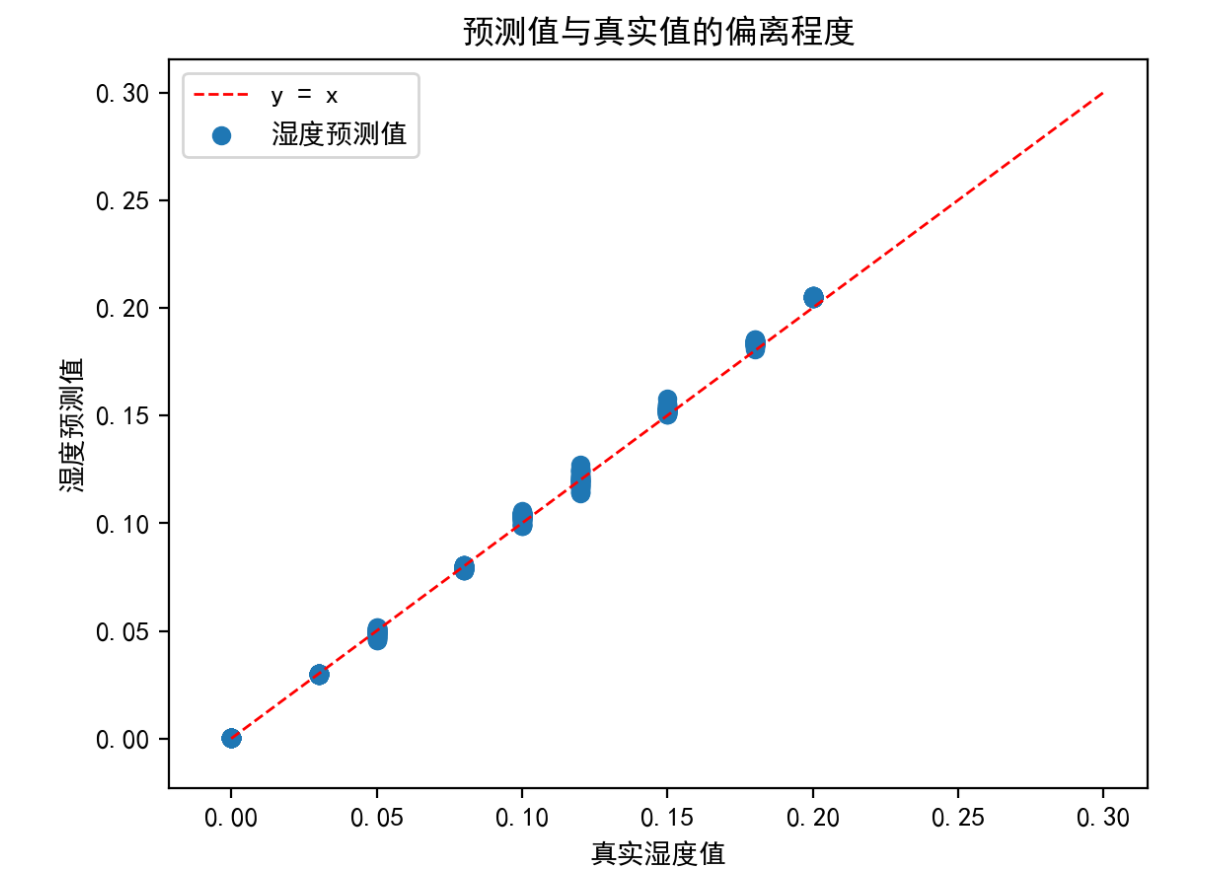其实神经网络这套方法都比较相似，机器的计算代替了很多人为的推理和演算。

希望可以和大家多多交流，共同进步(●'◡'●)！


展开全文深度学习 卷积
• 一维卷积神经网络在维度S上进行卷积 如下，设置一维卷积网络的输入通道为16维，输出通道为33维，卷积核大小为3，步长为2 # in_channels: 16 # out_channels: 33 # kernel_size: 3 m = nn.Conv1d(16, 33, 3, ...
设输入的数据维度是B x S x T

一维卷积神经网络在维度S上进行卷积

如下，设置一维卷积网络的输入通道为16维，输出通道为33维，卷积核大小为3，步长为2

# in_channels: 16
# out_channels: 33
# kernel_size: 3
m = nn.Conv1d(16, 33, 3, stride=2)
input = torch.randn(20, 16, 50)
output = m(input)
# shape of output is ([20, 33, 24])
# 最后一个维度:
# (50 - 3)/2 + 1 = 24
print(output.shape)

如上所述，输入维度为20 x 16 x 50

经过一维卷积后输出维度是20 x 33 x 24

第二个维度从16变为33，因为一维卷积输入通道为16，对应输入的第二个维度，一维卷积输出为33，对应输出的第二个维度

最后一个维度从50变为24，将参数带入公式[(n+2p-f) / s + 1]向下取整得到[(50-3)/2 + 1] = 24

而全连接神经网络对维度T进行卷积

使用和上述相同的输入维度，设置全连接神经网络的输入维度为input的最后一个维度50，输出维度为33

m1 = nn.Linear(50, 33)
output1 = m1(input)
# shape of output1 is ([20, 16, 33])
print(output1.shape)


将输入通过全连接神经网络后得到输出维度为20 x 16  x 33

即，全连接神经网络只在输入的最后一个维度进行卷积


展开全文卷积
• 一维卷积神经网络（1D CNNs）只在一定程度上有所涉及，比如在自然语言处理（NLP）中的应用。目前很少有文章能够提供关于如何构造一维卷积神经网络来解决你可能正面临的一些机器学习问题。本文试图补上这样一个短板...
• 我所采用的数据集，是我自己构建的网络流量数据集，借鉴了Wei Wang等人端到端的思想， 但是处理成的数据集却不同于他们的MNIST型数据集，而是采用的npy进行存储。 由于只是用于测试模型搭建，该数据集仅包含了...keras
• (8)卷积神经网络如何处理一维时间序列数据？ 概述 许多文章都关注于二维卷积神经网络（2D CNN）的使用，特别是图像识别。而一维卷积神经网络（1D CNNs）只在一定程度上有所涉及，比如在自然语言处理（NLP）中的...
• 基于一维卷积神经网络对机械振动信号进行分类并加以预测 *使用一维卷积神经网络训练振动信号进行二分类 2020年7月16日，一学期没等到开学，然而又放假了。 总览CSDN中大多数卷积神经网络都是对二维图片进行分类的，...tensorflow 深度学习 机器学习
• 文章目录基于一维卷积神经网络的滚动轴承故障识别一、数据预处理二、模型搭建三、使用步骤1.引入库2.读入数据总结 一、数据预处理 采用美国凯斯西储大学(CWRU) 的开放轴承数据库的样本进行实验分析，轴承故障产生的...深度学习
• 前面两篇文章介绍的是卷积神经网络处理二维数据，那么对于应用更多的一维时间序列数据，该如何用卷积神经网络来处理呢？概述许多文章都关注于二维卷积神经网络（2D CNN）的使用，特别是图像识别...
• 目的：针对传统机器学习算法对于流量分类的瓶颈问题，提出基于一维卷积神经网络模型的应用程序流量分类算法。 数据集：ICSX VPN-nonVPN 特征：数据预处理工具“USTC-TK2016， 本文选择同文献相同的前784 Byte，...
• 卷积神经网络的学习 https://www.cnblogs.com/szxspark/p/8439066.html
• 概述许多文章都关注于二维卷积神经网络（2D CNN）的使用，特别是图像识别。而一维卷积神经网络（1D CNNs）只在一定程度上有所涉及，比如在自然语言处理（NLP）中的应用。目前很少有文...
• 原文地址：Introduction to 1D Convolutional Neural Networks in Keras for Time Sequences 原文作者：Nils Ackermann 译文出自：掘金翻译计划 ...译者：haiyang-tju ...许多技术文章都关注于二卷积...
• Python-深度学习-学习笔记（13）：keras搭建卷积神经网络（对二维数据进行一维卷积） 卷积神经网络进行图像分类是深度学习关于图像处理的一个应用，卷积神经网络的优点是能够直接与图像像素进行卷积，从图像像素中...卷积 池化层 降维
• 基于1DCNN(一维卷积神经网络）的机械振动故障诊断 机械振动故障诊断最为经典的还是凯斯西储实验室的轴承故障诊断，开学一周了，上次改编鸢尾花分类的代码可用，但是并不准确。开学一周重新改编了别人的一篇代码，亲...深度学习 tensorflowTensorflow
• 1. 二卷积 ...如果你对卷积维度的计算不清楚，可以参考我之前的博客吴恩达深度学习笔记（deeplearning.ai）之卷积神经网络（CNN）（上）。 上述内容没有引入channel的概念，也可以说channel的数量为...二维
• ## 神经网络学习 之 BP神经网络

万次阅读 多人点赞 2015-11-30 21:17:56
次我们讲了M-P模型，它实际上就是对单个神经元的种建模，...本文具体来介绍一下种非常常见的神经网络模型——反向传播(Back Propagation)神经网络。概述BP（Back Propagation）神经网络是1986年由Rumelhart和Mc
• 一般来说，一维卷积用于文本数据，二维卷积用于图像数据，对宽度和高度都进行卷积，三维卷积用于视频及3D图像处理领域（检测动作及人物行为），对立方体的三个面进行卷积 。二维卷积的用处范围最广，在计算机视觉中...卷积 pytorch
• 4.4特殊应用:人脸识别和神经网络风格转换 觉得有用的话,欢迎一起讨论相互学习~Follow Me 4.11一维和三维卷积 二维和一维卷积 * 对于2D卷积来说，假设原始图像为14∗14∗314∗14∗314*14*3的三通道图像，...3D卷积
• ## 卷积神经网络

万次阅读 多人点赞 2014-11-29 16:20:41
自今年七月份以来，一直在实验室负责卷积神经网络（Convolutional Neural Network，CNN），期间配置和使用过theano和cuda-convnet、cuda-convnet2。为了增进CNN的理解和使用，特写此博文，以其与人交流，互有增益。...
• 在网上找资料的过程中，发现并没有特别细致的讲解分类图像和分类一维向量的做法，导致我捅咕了有几天才弄明白，可能使我比较菜吧......现在在这里记录一下。 首先需要明确，前文我们已经讲解了包装数据集的方法，...pytorch
• 点击蓝字关注我们AI TIME欢迎每位AI爱好者的加入！普通的神经网络不适用于比较大的网格（因为参数会过多），而传统的卷积神经网络又没法应用在拥有不规则结构的三网格上。因此，我们把传...卷积 网络 算法 编程语言
• 神经网络是一门重要的机器学习技术。它是目前最为火热的研究方向--深度学习的基础。学习神经网络不仅可以让你掌握一门强大的机器学习方法...　神经网络种模拟人脑的神经网络以期能够实现类人工智能的机器学习技...
• 在这里给大家分享篇关于用深度学习...这篇文章是由韩国的几个研究人员发表的，通过使用卷积神经网络实现了心电图的高精准分类，总共完成了8类心电图心律不齐病变的分类，其达到的分类精确度都超过了所有目前用来...人工智能
• 我在写CupCnn的时候，个困扰我很久的问题，就是如何组织卷积神经网络数据结构。尤其是卷积层和全连接层之间的衔接问题。卷积层至少需要四数据结构（batch+channel+height+width）,而全连接层则只需个二...卷积 java
• ## 卷积神经网络CNN：一维信号输入

万次阅读 多人点赞 2018-08-30 11:22:25
时光荏苒，转眼间已经过去两个月了，这段日子大概是我研究生生涯中最忙碌的段时光，从个python小白，深度学习小白，到现在感觉自己总算摸到了深度学习的门槛，心中还是有一些欣慰和感慨，可能对于很多人来说，我......